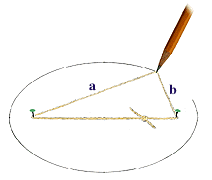# EllipsesThe paths the planets describe are ellipses, though some orbits are nearly circular. An ellipse is defined as a closed plane curve generated when a point, P, moves in a path such that the sum of the distances from two fixed points is a constant. The two fixed points are the foci of the ellipse. For the orbits of the planets, the Sun is at one focus.

One way to draw an ellipse makes use of the notion that the "sum of the distances from two fixed points is a constant." Using two tacks as the foci, loop a string around them, then place a pencil in the loop to pull it taut and move the point around the loop. The path describes an ellipse. Since the string cannot grow or shrink in length, the sum of the distances from the two tacks (a + b) is a constant.

The eccentricity of an ellipse is a measure of its elongation, found by dividing the distance between the foci by the length of the major axis. This number is between 0 and 1 -- the larger the value, the more elongated the ellipse. (A circle has an eccentricity of 0.) Pluto's orbit is very elliptical, with an eccentricity of 0.25, the highest of all the planets.

Back to Kepler's Laws